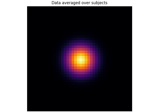# mne.stats.f_oneway#

mne.stats.f_oneway(*args)[source]#

Perform a 1-way ANOVA.

The one-way ANOVA tests the null hypothesis that 2 or more groups have the same population mean. The test is applied to samples from two or more groups, possibly with differing sizes .

This is a modified version of `scipy.stats.f_oneway()` that avoids computing the associated p-value.

Parameters:
*argsarray_like

The sample measurements should be given as arguments.

Returns:
F-value`float`

The computed F-value of the test.

Notes

The ANOVA test has important assumptions that must be satisfied in order for the associated p-value to be valid.

1. The samples are independent

2. Each sample is from a normally distributed population

3. The population standard deviations of the groups are all equal. This property is known as homoscedasticity.

If these assumptions are not true for a given set of data, it may still be possible to use the Kruskal-Wallis H-test (`scipy.stats.kruskal()`) although with some loss of power.

The algorithm is from Heiman , pp.394-7.

References

## Examples using `mne.stats.f_oneway`#Statistical inference

Statistical inference